# C++中 vector容器——模板类数组

对vector容器的一点理解，相对于数组，vector容器可以不固定大小，但是需要而外的内存

空间，这是用空间来换取数组动态大小的一种方式

1.定义

vector <int> A;   定义了一个空的int型数组

vector<double> B(n); 定义了一个长度为n的double型数组

vector<int> B(C);  定义一个C的副本

vector<int> D(n,i); 定义并初始化D,为n个i元素

2.基本的操作

一. 插入操作

尾部插入   A.push_back(i);  把元素i插入A的尾部

指定位置插入 A.insert(p,i)   在迭代器p前插入元素i

二.删除操作

A.erase(p);  删除迭代器p所指向的元素

A.pop_back(); 弹出最后一个元素，并不会返回

三.遍历

通过下标(和数组一样）

通过迭代器遍历

``````#include<iostream>
#include<vector>
using namespace std;
int main()
{
int i;
vector<int> A;
for(i=0;i<5;i++)
A.push_back(2*i+1);

for(i=0;i<5;i++) //利用下标遍历
cout<<A[i]<<" ";

cout<<endl;

vector<int>::iterator it; //定义了一个迭代器
for(it=A.begin();it!=A.end();it++)  //利用迭代器遍历
cout<<*it<<" ";

cout<<endl;

for(i=0;i<5;i++)
cout<<A.at(i)<<" ";
}
``````

##### 热门推荐
•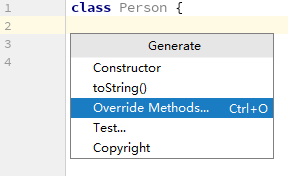浏览(649)
•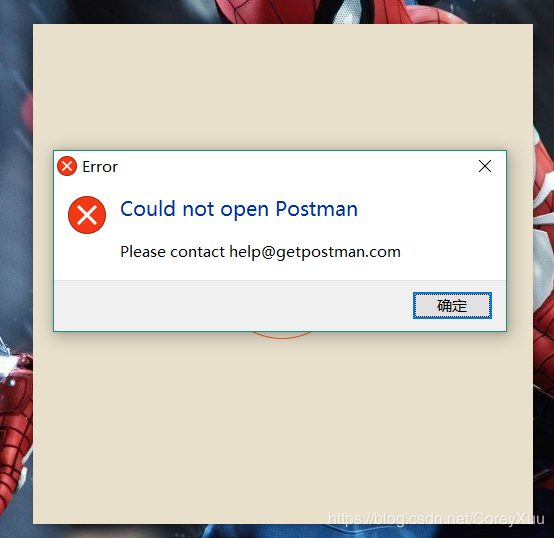浏览(556)
•浏览(540)
•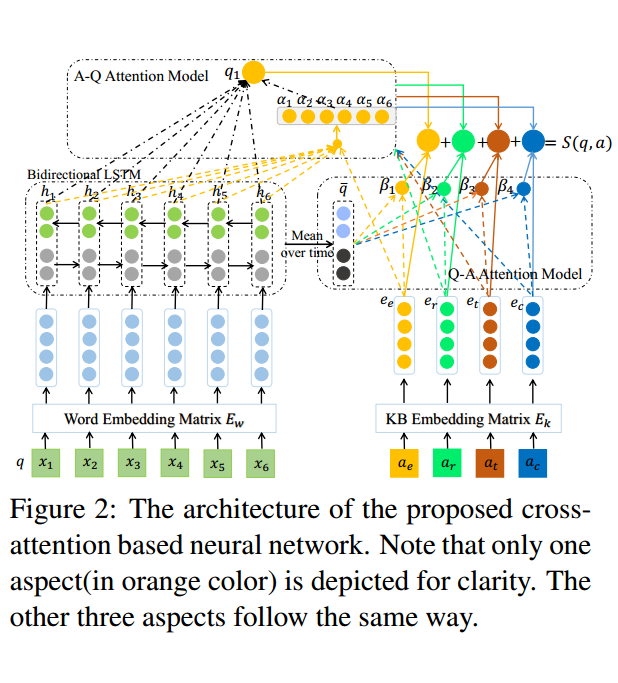浏览(468)
•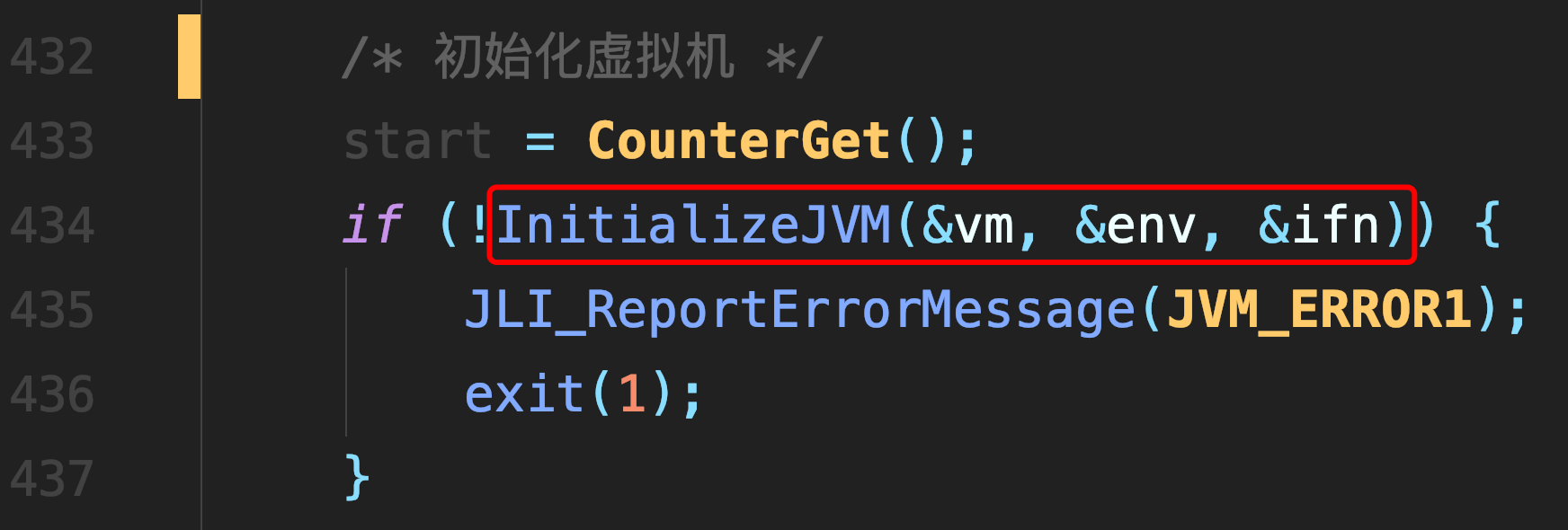浏览(423)
•浏览(402)
•浏览(379)
•浏览(366)
•浏览(357)
•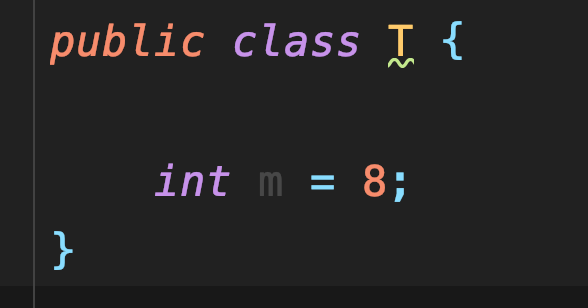浏览(315)